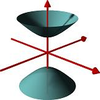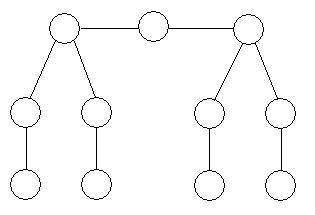#### You may also like### A Close Match

Can you massage the parameters of these curves to make them match as closely as possible?### Prime Counter

A short challenge concerning prime numbers.### The Right Volume

Can you rotate a curve to make a volume of 1?

# Numbered Graph

##### Age 16 to 18Challenge Level

A numbered graph consists of a set of numbered nodes connected by edges. The node sum at a node is the sum of the number at the node and the numbers at all adjacent nodes.

Can you arrange the numbers 1 to 11 on the graph below, using each number exactly once, so the the node sum is the same at each node?Did you know ... ?

The notion of the mathematical graph is fundamental in modern mathematics and a huge subject of study in its own right. Many systems can be represented by graphs, the internet being a somewhat complicated example. Graph theory is both a fascinating area of pure study and a powerful analytical tool.